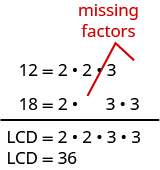## Adding and Subtracting Fractions With Different Denominators

### Learning Outcomes

• Add or subtract fractions with different denominators
• Add or subtract fractions that contain variables and have different denominators
• Identify and use fraction operations

Once we have converted two fractions to equivalent forms with common denominators, we can add or subtract them by adding or subtracting the numerators.

### Add or subtract fractions with different denominators

1. Find the LCD.
2. Convert each fraction to an equivalent form with the LCD as the denominator.
3. Add or subtract the fractions.
4. Write the result in simplified form.

### Example

Add: $\Large\frac{1}{2}+\Large\frac{1}{3}$

Solution:

 $\Large\frac{1}{2}+\Large\frac{1}{3}$ Find the LCD of $2$, $3$.Change into equivalent fractions with the LCD $6$. $\Large\frac{1\cdot\color{red}{3}}{2\cdot\color{red}{3}} +\Large\frac{1\cdot\color{red}{2}}{3\cdot\color{red}{2}}$ Simplify the numerators and denominators. $\Large\frac{3}{6}+\Large\frac{2}{6}$ Add. $\Large\frac{5}{6}$

Remember, always check to see if the answer can be simplified. Since $5$ and $6$ have no common factors, the fraction $\Large\frac{5}{6}$ cannot be reduced.

### Try It

Watch the following video to see more examples and explanation about how to add two fractions with unlike denominators.

### Example

Subtract: $\Large\frac{1}{2}-\left(-\Large\frac{1}{4}\right)$

### Try It

The following video provides two more examples of how to subtract two fractions with unlike denominators.

### Example

Add: $\Large\frac{7}{12}+\Large\frac{5}{18}$

### Try It

When we use the Equivalent Fractions Property, there is a quick way to find the number you need to multiply by to get the LCD. Write the factors of the denominators and the LCD just as you did to find the LCD. The “missing” factors of each denominator are the numbers you need.The LCD, $36$, has $2$ factors of $2$ and $2$ factors of $3$.

Twelve has two factors of $2$, but only one of $3$ —so it is ‘missing‘ one $3$. We multiplied the numerator and denominator of $\Large\frac{7}{12}$ by $3$ to get an equivalent fraction with denominator $36$.

Eighteen is missing one factor of $2$ —so you multiply the numerator and denominator $\Large\frac{5}{18}$ by $2$ to get an equivalent fraction with denominator $36$. We will apply this method as we subtract the fractions in the next example.

### Example

Subtract: $\Large\frac{7}{15}-\Large\frac{19}{24}$

### Example

Add: $-\Large\frac{11}{30}+\Large\frac{23}{42}$

### Try It

In the next example, one of the fractions has a variable in its numerator. We follow the same steps as when both numerators are numbers.

### Example

Add: $\Large\frac{3}{5}+\Large\frac{x}{8}$

### Try It

Watch the following video to see more examples of how to add and subtract fractions with unlike denominators that contain variables.